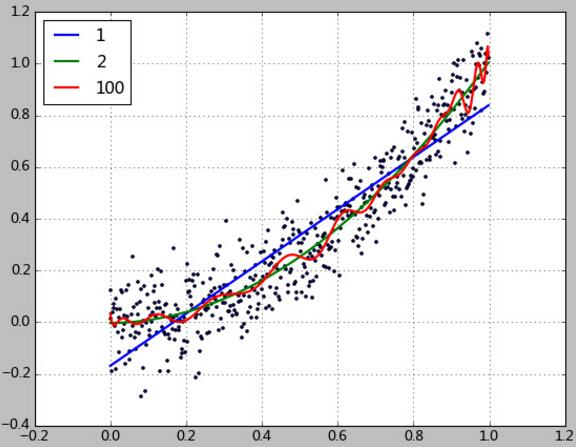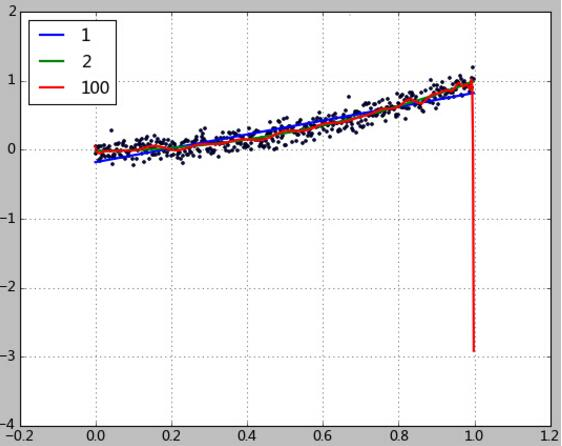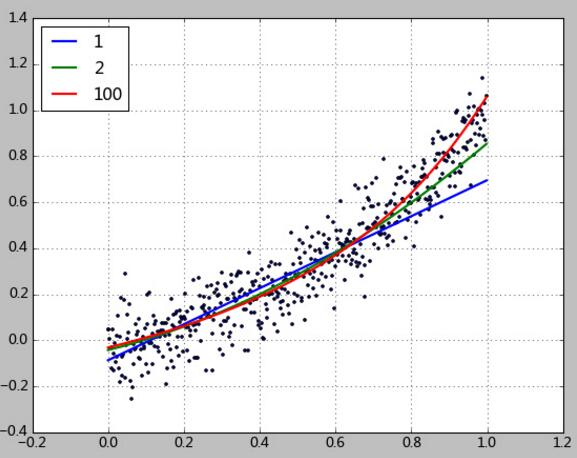# Python数据拟合与广义线性回归算法学习

更新时间：2017年12月22日 10:37:36   作者：lsldd我要评论

```import matplotlib.pyplot as plt
import numpy as np
import scipy as sp
from scipy.stats import norm
from sklearn.pipeline import Pipeline
from sklearn.linear_model import LinearRegression
from sklearn.preprocessing import PolynomialFeatures
from sklearn import linear_model

''''' 数据生成 '''
x = np.arange(0, 1, 0.002)
y = norm.rvs(0, size=500, scale=0.1)
y = y + x**2

''''' 均方误差根 '''
def rmse(y_test, y):
return sp.sqrt(sp.mean((y_test - y) ** 2))

''''' 与均值相比的优秀程度，介于[0~1]。0表示不如均值。1表示完美预测.这个版本的实现是参考scikit-learn官网文档 '''
def R2(y_test, y_true):
return 1 - ((y_test - y_true)**2).sum() / ((y_true - y_true.mean())**2).sum()

''''' 这是Conway&White《机器学习使用案例解析》里的版本 '''
def R22(y_test, y_true):
y_mean = np.array(y_true)
y_mean[:] = y_mean.mean()
return 1 - rmse(y_test, y_true) / rmse(y_mean, y_true)

plt.scatter(x, y, s=5)
degree = [1,2,100]
y_test = []
y_test = np.array(y_test)

for d in degree:
clf = Pipeline([('poly', PolynomialFeatures(degree=d)),
('linear', LinearRegression(fit_intercept=False))])
clf.fit(x[:, np.newaxis], y)
y_test = clf.predict(x[:, np.newaxis])

print(clf.named_steps['linear'].coef_)
print('rmse=%.2f, R2=%.2f, R22=%.2f, clf.score=%.2f' %
(rmse(y_test, y),
R2(y_test, y),
R22(y_test, y),
clf.score(x[:, np.newaxis], y)))

plt.plot(x, y_test, linewidth=2)

plt.grid()
plt.legend(['1','2','100'], loc='upper left')
plt.show() ```[-0.16140183  0.99268453]
rmse=0.13, R2=0.82, R22=0.58, clf.score=0.82
[ 0.00934527 -0.03591245  1.03065829]
rmse=0.11, R2=0.88, R22=0.66, clf.score=0.88
[  6.07130354e-02  -1.02247150e+00   6.66972089e+01  -1.85696012e+04
......
-9.43408707e+12  -9.78954604e+12  -9.99872105e+12  -1.00742526e+13
-1.00303296e+13  -9.88198843e+12  -9.64452002e+12  -9.33298267e+12
-1.00580760e+12]
rmse=0.10, R2=0.89, R22=0.67, clf.score=0.89

y = 0.99268453x -0.16140183

1、误差分析。

RMSE是预测值与真实值的误差平方根的均值。这种度量方法很流行（Netflix机器学习比赛的评价方法），是一种定量的权衡方法。
R2方法是将预测值跟只使用均值的情况下相比，看能好多少。其区间通常在（0,1）之间。0表示还不如什么都不预测，直接取均值的情况，而1表示所有预测跟真实结果完美匹配的情况。
R2的计算方法，不同的文献稍微有不同。如本文中函数R2是依据scikit-learn官网文档实现的，跟clf.score函数结果一致。

2、过拟合。

clf.fit(x[:498, np.newaxis], y[:498])[-0.17933531  1.0052037 ]
rmse=0.12, R2=0.85, R22=0.61, clf.score=0.85
[-0.01631935  0.01922011  0.99193521]
rmse=0.10, R2=0.90, R22=0.69, clf.score=0.90
...
rmse=0.21, R2=0.57, R22=0.34, clf.score=0.57

clf = Pipeline([('poly', PolynomialFeatures(degree=d)),
('linear', linear_model.Ridge ())])
clf.fit(x[:400, np.newaxis], y[:400])[ 0.          0.75873781]
rmse=0.15, R2=0.78, R22=0.53, clf.score=0.78
[ 0.          0.35936882  0.52392172]
rmse=0.11, R2=0.87, R22=0.64, clf.score=0.87
[  0.00000000e+00   2.63903249e-01   3.14973328e-01   2.43389461e-01
1.67075328e-01   1.10674280e-01   7.30672237e-02   4.88605804e-02
......
3.70018540e-11   2.93631291e-11   2.32992690e-11   1.84860002e-11
1.46657377e-11]
rmse=0.10, R2=0.90, R22=0.68, clf.score=0.90